# Milne problem

A problem in radiative transfer theory concerning the single-velocity kinetic transport equation of quanta or particles in a half-space. The integral equation of the Milne problem with a source at infinity under zero incident flux of the radiation was first introduced by E. Milne  for the case of isotropic scattering of quanta, diffusing without absorption in a stellar atmosphere.

The Milne equation takes the form(1)

Hereis the radiation (or particle) density and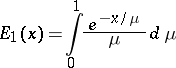is the exponential integral function.

In neutron physics the Milne problem is used for the formulation of approximate boundary conditions for solutions of the equations of a diffusion approximation in a bounded domain; in this connection one takes into account neutron capture by the medium, anisotropic scattering and the curvature of the boundary.

Here the Milne problem is to solve the integro-differential equation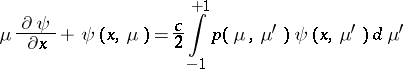with boundary conditions on the boundary of the half-space occupied by the matter, with a vacuum(2)

whereis the mean number of secondary neutrons, colliding once with a nucleus (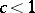for scattering and absorbing neutrons by the medium), and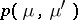is the indicatrix of the scattering (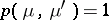for isotropic scattering). The spherical or cylindrical Milne problem on the distribution of neutrons in the space outside absorbing spheres or cylinders is stated analogously.

The solution of the Milne problem is conveniently given by applying the Laplace transform to the integro-differential transfer equation (see ) and using the Wiener–Hopf method to solve the functional equations thus obtained.

In order to solve the Milne problem it was suggested that expansion relative to generalized eigen functions and methods for solving singular integral equations be used (see ). The solution of the Milne problem for,is sought in the formwhere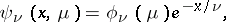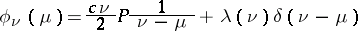are the eigen functions of the continuous spectrum,denotes the Cauchy principal value,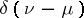is the Dirac-function, and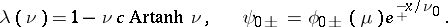The discrete eigen valuesare the roots of the characteristic equation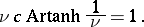The eigen functions of the discrete spectrum take the form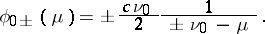The system of eigen functionsand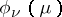,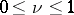, turns out to be complete in the space of generalized functions on the interval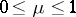and they are orthogonal with respect to a weight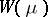which is the solution of a singular integral equation (see ).

The boundary condition (2) of the Milne problem gives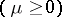: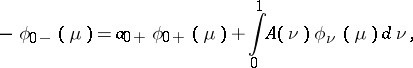that is,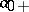and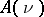are defined as the coefficients in the expansion of the function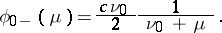The asymptotic density of the neutrons,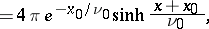vanishes for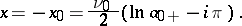For,, the Hopf constant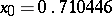.